### Vibrations of Continuous Systems: Lateral Vibrations of Beams

### Equation of Motion

Consider a beam, shown in Figure 10.6(a) which has a length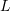, density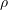(mass per unit volume) and Young’s modulus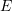which is acted upon by a distributed load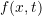(per unit length) acting laterally along the beam. Let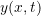measure the lateral deflection of the beam and assume that only small deformations occur. Figure 10.6(b) shows the FBD/MAD for an infinitesimal element of the beam with mass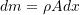. By applying Newton’s Law’s and considering moments about the left end of the element we find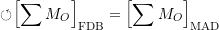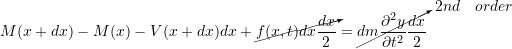As a first order approximation we can write that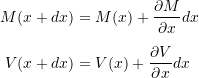so that the above becomes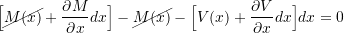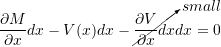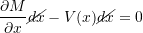or

(10.23)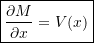which is a well known result from beam theory. In the vertical direction we then have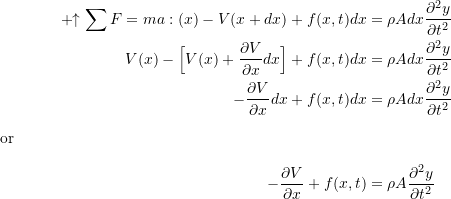Note: For small motions, the shear forces act vertically to a first order approximation. The actual vertical components would be for example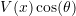where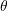can be obtained from the slope of the beam. However, for small motions,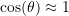, so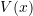is used here as the vertical component. A similar remark holds for the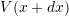term.

Now, using 10.23 we can write that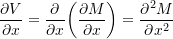so the equation of motion becomes

(10.24)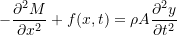For small deformations the bending moment in the beam is related to the deflection by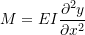so that 10.24 becomes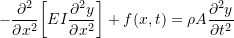or

(10.25)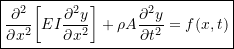This is the general equation which governs the lateral vibrations of beams. If we limit ourselves to only consider free vibrations of uniform beams (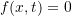,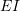is constant), the equation of motion reduces to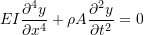which can be written

(10.26)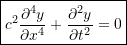where

(10.27)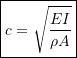Note that this is not the wave equation.

### Solution To Equation of Motion

Once again we look for solutions which represent a mode shape undergoing \sshm of the form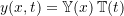This results in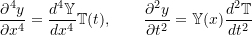so 10.26 becomes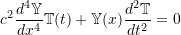Separating theandterms we find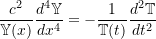Again, since the LHS depends only onand the RHS depends only onand they must be equal for all values ofand, both sides must be equal to a constant, which we call, so that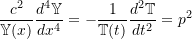This results in equations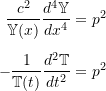or

(10.28a)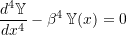(10.28b)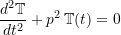where

(10.29)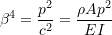Note for future reference that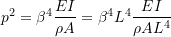so that

(10.30)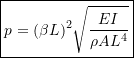The solution to 10.28b is as we have seen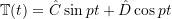To find the solution to 10.28a, which is a 4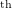order linear ODE with constant coefficients, we assume a solution of the form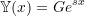The equation of motion then becomes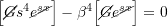or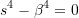The four roots to this equation are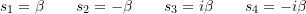The total solution will be a linear combination of solutions, one for each of the above roots,

(10.31)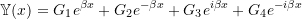where each of the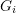may be complex. However, by using Euler’s identity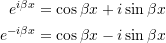and introducing the hyperbolic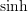and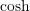functions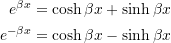10.31 can be written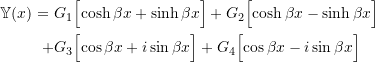or

(10.32)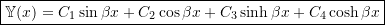where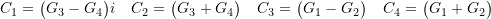The advantage of expressing the solution as in 10.32 is that all of the terms involved are real. We see that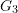and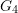are complex conjugates, while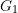and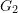are real.

As can be seen there are four constants to be determined which requires that four boundary conditions be specified. These will often be determined with one pair of boundary conditions specified at each end, depending on the type of support. Figure 10.7 shows three of the most common support conditions and the pairs of boundary conditions that are associated with each type of support.

#### EXAMPLE

A uniform beam (density, cross–sectional area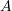, flexural rigidity) of lengthis fixed at one end and free at the other.

Determine expressions for the natural frequencies and associated mode shapes for this beam.

#### Complete Response of Lateral Motion of Beam

We have again found an infinite number of solutions which satisfy the equation of motion 10.26 given by

(10.33)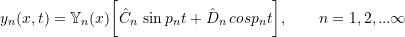where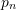is the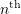natural frequency andis the associated mode shape, both of which depend on the specific boundary conditions present. The general solution will then be a superposition of all of the solutions in 10.33,

(10.34)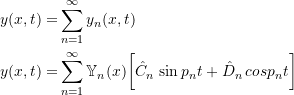The constants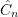and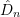are to be determined from the initial conditions of the beam. If the initial displacement and velocity of the beam are specified as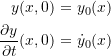then 10.34 gives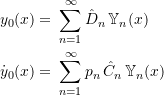andcan then be found from

(10.35)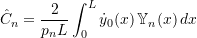(10.36)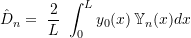where once again the orthogonality property of the mode shape has been used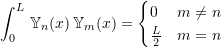This is once again beyond the scope of this course.